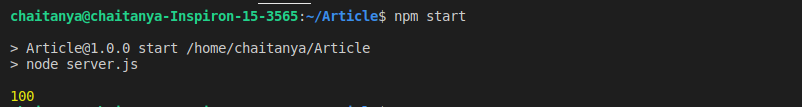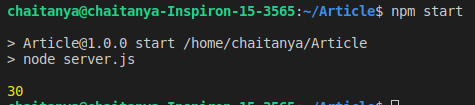# How to write asynchronous function for Node.js ?

The asynchronous function can be written in Node.js using ‘async’ preceeding the function name. The asynchronous function returns implicit Promise as a result. The async function helps to write promise based code asynchronously via the event-loop. Async functions will always return a value. Await function can be used inside asynchronous function to wait for the promise. This forces the code to wait until the promise returns a result.

Install async from npm in Node.js using the following command:

`npm i async`

Use async inside your Node.js project using require() method.

Example 1: Create asynchronous function to calculate square of a number inside Node.js.

• Create a project folder.
• Use the following command to initialize the package.json file inside the project folder.
`npm init -y`
• Install async using the following command:
`npm i async`
• Create a server.js file & write the following code inside it.
• Run the code using `npm start`

 `var` `async = require(``"async"``); ` ` `  `function` `square(x) { ` `    ``return` `new` `Promise(resolve => { ` `        ``setTimeout(() => { ` `            ``resolve(Math.pow(x, 2)); ` `        ``}, 2000); ` `    ``}); ` `} ` ` `  `async ``function` `output(x) { ` `    ``const ans = square(x); ` `    ``console.log(ans); ` `} ` ` `  `output(10); `

Output:Example 2: Create an asynchronous function to calculate the sum of two numbers inside Node.js using await. Perform the above procedure to create a Node.js project.

 `var` `async = require(``"async"``); ` ` `  `function` `square(a, b) { ` `    ``return` `new` `Promise(resolve => { ` `        ``setTimeout(() => { ` `            ``resolve(a + b); ` `        ``}, 2000); ` `    ``}); ` `} ` ` `  `async ``function` `output(a, b) { ` `    ``const ans = await square(a, b); ` `    ``console.log(ans); ` `} ` ` `  `output(10, 20); `

Output:My Personal Notes arrow_drop_upCheck out this Author's contributed articles.

If you like GeeksforGeeks and would like to contribute, you can also write an article using contribute.geeksforgeeks.org or mail your article to contribute@geeksforgeeks.org. See your article appearing on the GeeksforGeeks main page and help other Geeks.

Please Improve this article if you find anything incorrect by clicking on the "Improve Article" button below.

Article Tags :

Be the First to upvote.

Please write to us at contribute@geeksforgeeks.org to report any issue with the above content.